RRB Group D Mock Test - 12

# RRB Group D Mock Test - 12

Test Description

## 100 Questions MCQ Test RRB (Group D) - Mock Tests & Previous Year Papers | RRB Group D Mock Test - 12

RRB Group D Mock Test - 12 for Railways 2023 is part of RRB (Group D) - Mock Tests & Previous Year Papers preparation. The RRB Group D Mock Test - 12 questions and answers have been prepared according to the Railways exam syllabus.The RRB Group D Mock Test - 12 MCQs are made for Railways 2023 Exam. Find important definitions, questions, notes, meanings, examples, exercises, MCQs and online tests for RRB Group D Mock Test - 12 below.
Solutions of RRB Group D Mock Test - 12 questions in English are available as part of our RRB (Group D) - Mock Tests & Previous Year Papers for Railways & RRB Group D Mock Test - 12 solutions in Hindi for RRB (Group D) - Mock Tests & Previous Year Papers course. Download more important topics, notes, lectures and mock test series for Railways Exam by signing up for free. Attempt RRB Group D Mock Test - 12 | 100 questions in 90 minutes | Mock test for Railways preparation | Free important questions MCQ to study RRB (Group D) - Mock Tests & Previous Year Papers for Railways Exam | Download free PDF with solutions
 1 Crore+ students have signed up on EduRev. Have you?
RRB Group D Mock Test - 12 - Question 1

### A father is 3 yrs more than thrice the age of his son. Three yrs later, the father will be 10 yrs more than twice the age of his son. The ratio of their ages is

Detailed Solution for RRB Group D Mock Test - 12 - Question 1

Let present age of the son = x.
Ten present age of the father = 3x + 3
After three years, age of the son = (x+3) and age of the father = (3x+3+3) = (3x+6)
Given that (3x+6) = 10 + 2(x+3)
⇒ 3x + 6 = 10 + 2x + 6
⇒ x = 10
Present age of the father = 3x+3 = 3x10 + 3 = 33
ratio = 33 : 10

RRB Group D Mock Test - 12 - Question 2

### The values of x and y satisfying the given equations ax + by = 2 and abxy = 1 are respectively

Detailed Solution for RRB Group D Mock Test - 12 - Question 2 Since x = a-1 and
y = b-1
Satisfied both the equation ax + by = 2
abxy = 1
ax + by = a.a-1 + b.b-1 = 1 + 1 = 2
abxy = aba-1b-1 = 1
RRB Group D Mock Test - 12 - Question 3

### Which state has the lowest population growth rate?

RRB Group D Mock Test - 12 - Question 4

The average of five numbers is 27. If one number is excluded, the average becomes 25. The excluded number is :

Detailed Solution for RRB Group D Mock Test - 12 - Question 4

Average of 5 numbers = 27
∴ Sum of 5 numbers = 27 x 5 = 135
Given one number is excluded and average of remaining 4 numbers = 25
∴ Sum of remaining 4 numbers = 25 x 4 = 100
∴ Excluded Number = 135 - 100 = 35

RRB Group D Mock Test - 12 - Question 5

The wheel of a scooter has a diameter of 70 cm. The number revolution it must make to maintain a speed of 33 km/hour is

Detailed Solution for RRB Group D Mock Test - 12 - Question 5

The distance covered by the wheel in one m = 33 x 1000 x 100/60 = 55000
The distance covered by the wheel in one revolution
= The circumference of the wheel = 2πr
= 2 x 22/7 x 70/2 = 220cm
∴ Number of revolutions of the wheel = 55000/220 = 250

RRB Group D Mock Test - 12 - Question 6
A river is flowing at a speed of 5 km/hr in a particular direction. A man, who can swim at a speed of 20 km/hr in still water, Starts swimming along the direction of flow of the river from point A and reaches another point B which is at a distance of 30 km from the starting point A. On reaching point B, the man turns back and starts swimming against the direction of flow of the river and stops after reaching point A. The total time taken by the man to complete his journey is
RRB Group D Mock Test - 12 - Question 7
Who among the following was the author of 'Ashtadhyayi'?
RRB Group D Mock Test - 12 - Question 8
Pointing to a photograph a woman said, "She is the only daughter of my mother's father". How is the woman related to the person in the photograph?
RRB Group D Mock Test - 12 - Question 9
Choose the analogous pair.
Subpoena : Witness
RRB Group D Mock Test - 12 - Question 10
Choose the word which is least like the other words in the group .
RRB Group D Mock Test - 12 - Question 11
In a coding system PEN is written as NZO and BARK as DTSL. How can we write PRANK in that system ?
RRB Group D Mock Test - 12 - Question 12
Complete the analogous pair.
Robert Hooks : Robert Brown : : Cells : ?
RRB Group D Mock Test - 12 - Question 13

A sum of money lent out at simple interests amounts to Rs. 720 after 2 years and to Rs. 1020 after a further period of 5 years. The sum is:

Detailed Solution for RRB Group D Mock Test - 12 - Question 13

Principal + SI for 2 years = Rs 720 ……(I)
Principal + SI for 7 years = Rs 1020 ……(II)
Subtracting equation (I) from (II),
S.I. for 5 years = (1020 – 720) = Rs 300
∴ S.I. for 2 years = 300 x 2/5 = Rs 120
∴ Principal = (720 – 120) = Rs 600

RRB Group D Mock Test - 12 - Question 14
Find the number of triangles in the given figure.
RRB Group D Mock Test - 12 - Question 15
Which two countries are connected by underwater tunnel recently constructed?
RRB Group D Mock Test - 12 - Question 16
Three position of a dice are shown below

Which number is opposite to 6?
RRB Group D Mock Test - 12 - Question 17
Which country's currency code is AUD?
RRB Group D Mock Test - 12 - Question 18
Apart from India, which one of the following countries celebrates its Independence Day on the same day, August 15?
RRB Group D Mock Test - 12 - Question 19
Who is the author of the book 'A Foreign Policy of India'?
RRB Group D Mock Test - 12 - Question 20
A man travels 3 kms to the West, turns left and goes 3 kms, turns right and goes 1 km, again turns right and goes 3 kms. How far is he from the starting point?
RRB Group D Mock Test - 12 - Question 21
The Reserve Bank of India (RBI) has recently unveiled new Rs 10 denomination banknote under the Mahatma Gandhi (New) Series. The new note has the motif of______
RRB Group D Mock Test - 12 - Question 22
According to the latest statistics of the government, what percent of growth rate has been registered by the banking sector in December 2017 post Centre’s PSB recapitalisation plan?
RRB Group D Mock Test - 12 - Question 23
'Indian cotton merchant; banker ; Congressman and a close associate of Mahatma Gandhi'. This description fits
RRB Group D Mock Test - 12 - Question 24
The first Indian University was opened in 1857 was in
RRB Group D Mock Test - 12 - Question 25
MICR stands for
RRB Group D Mock Test - 12 - Question 26
Thrashing is caused by
RRB Group D Mock Test - 12 - Question 27
Which of the following is the leading export item among Indian Plantaion Crops?
RRB Group D Mock Test - 12 - Question 28
National Sample Survey (NSS) was established in—
RRB Group D Mock Test - 12 - Question 29
Jawaharlal Nehru Port is a satellite port of -
RRB Group D Mock Test - 12 - Question 30
The Treaty of Alinagar was concluded between
RRB Group D Mock Test - 12 - Question 31
Which continent contributes maximum to rice output of the world?
RRB Group D Mock Test - 12 - Question 32
Where is the Metei language predominantly spoken?
RRB Group D Mock Test - 12 - Question 33
Consider the following statements :
The pitch of the sound produced by a stringed musical instrument can be increased by
1. Decreasing the length of the string.
2. Increasing the tension of the string.
3. Increasing the length of the string.
4. Decreasing the tension of the string.
Which of these statements is/are correct?
RRB Group D Mock Test - 12 - Question 34
Tsunamis are huge sea waves caused by
RRB Group D Mock Test - 12 - Question 35
Which part of the brain controls respiration?
RRB Group D Mock Test - 12 - Question 36
In human body, the role of bile salts in digestion is to
RRB Group D Mock Test - 12 - Question 37
Of the four landmarks in medical history given below, which one was the first to take place?
RRB Group D Mock Test - 12 - Question 38
"By weaving threads of physics, chemistry and biology into a rich tapestry, this remarkable scientist provided a unifying molecular view of the world. He touted the wounderful properties of Vitamin C first as a cure of common cold and later as a preventive agent against cancer." One of science's major figures of all time referred to above is
RRB Group D Mock Test - 12 - Question 39
Which one of the following is used as an anti-freeze the automobile engines?
RRB Group D Mock Test - 12 - Question 40
In the eye, donation, which part of the eye is transplanted from the donor?
RRB Group D Mock Test - 12 - Question 41
A girl is swimming on a swing in sitting position. When the same girl stands up, the period of swing will
RRB Group D Mock Test - 12 - Question 42
Cryogenic enciphers find applications in
RRB Group D Mock Test - 12 - Question 43
Which of the following professional (s) are more likely to run the risk of a permanent change in their cell's DNA?
1. Researches using carbon- 14 isotope
2. X-ray technician
3. Coal miner
4. Dyer and painter
Select the correct answer by using the codes given below:
RRB Group D Mock Test - 12 - Question 44
Arteries supplying blood to the heart are called
RRB Group D Mock Test - 12 - Question 45

India exchanged the list of nuclear installations and facilities with which country, simultaneously through diplomatic channels?

RRB Group D Mock Test - 12 - Question 46
Find the missing character.
Detailed Solution for RRB Group D Mock Test - 12 - Question 46 In the first column, 17-11=25-19=6.
In the second column, 12-6=34-28=6.
Let the missing number in the third column be x.
Then, x-8=19-11=8 or x=16.
RRB Group D Mock Test - 12 - Question 47

Let X be any point within a square ABCD. On AX a square AXYZ is described such that D is within it. Which one of the following is correct?

Detailed Solution for RRB Group D Mock Test - 12 - Question 47

AB = AD (side of a square ABCD)
and AX = AZ (side of a square AXYZ)
Let ∠BAX = θ
∴ ∠XAD = 90o - θ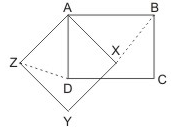Also, AXYZ is a square
∴ ∠ZAX = 90o
⇒ ∠ZAD = 90o - (90o - θ) = θ
∴ BX = DZ (by CPCT)

RRB Group D Mock Test - 12 - Question 48
Which of the following state/states permit the sale of cannabis for recreational use?
RRB Group D Mock Test - 12 - Question 49
Alexander Fleming discovered
RRB Group D Mock Test - 12 - Question 50
If in the word NOLOCONITENDERE all letters falling at even-number, positions are replaced by the digit 3 and all those falling at odd-number positions by 7, what will be the sum total of all 3's and all 7's in the entire word ?
RRB Group D Mock Test - 12 - Question 51
What is the missing element in the sequence represented by the question mark?
A, D, H, M, ?, Z
Detailed Solution for RRB Group D Mock Test - 12 - Question 51
RRB Group D Mock Test - 12 - Question 52

A survey was conducted on a samples of 1000 persons with reference to their knowledge of English, French and German. The result of the survey are presented in the given Venn diagram. The ratio of the number of the persons who do not know any of the three languages to those who know all the three languages, is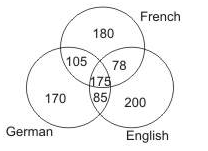Detailed Solution for RRB Group D Mock Test - 12 - Question 52

No. of people who do not know any of the three languages
= 1000 - (170 + 105 + 180 + 175 + 85 + 78 + 200)
= 1000 - 993 = 7
∴ Required ratio = 7/175 = 1/25

RRB Group D Mock Test - 12 - Question 53
Arrange the following in a meaningful order:
1. Birth
2. Death
3. Funeral
4. Marriage
5. Education
RRB Group D Mock Test - 12 - Question 54

If 'x' means '+', '+' means '÷', '÷' means '-' and '-' means 'x', what will be the value of the following expression?

5 - 7 x 9 + 3 ÷ 2

Detailed Solution for RRB Group D Mock Test - 12 - Question 54

5 - 7 x 9 + 3 ÷ 2
⇒ 5 x 7 + 9 ÷ 3 - 2 = 35 + 3 - 2
⇒ 38 - 2 = 36

RRB Group D Mock Test - 12 - Question 55

Milk and water are in the ratio of 3:2 in a mixture of 80 litres. How much water should be added so that the ratio of the milk and water becomes 2:3?

Detailed Solution for RRB Group D Mock Test - 12 - Question 55

Quantity of milk = (80 x 3/5 ) = 48 liters
Quantity of water in it = 80 - 48 = 32 liters
New ratio = 2 : 3
Let quantity of water to be added further be x litres
Then milk : water = 48/32 + x
Now, 48 /32 + x = 2/3
⇒ 64 + 2x = 144
⇒ 2x = 144 - 64 = 80⇒ x = 80/2 = 40

RRB Group D Mock Test - 12 - Question 56
Recently the Union Cabinet has approved the establishment of an AIIMS facility in which district of Himachal Pradesh at an outlay of Rs 1,351 crore?
RRB Group D Mock Test - 12 - Question 57
Name the state which has imposed complete ban on electronic cigarettes in the state with a jail term of up to 3 years on its violation
RRB Group D Mock Test - 12 - Question 58
Complete the analogous pair.
211 : 333 :: 356 : ?
Detailed Solution for RRB Group D Mock Test - 12 - Question 58 Addition of sum of digits of 2nd term (333) and 3rd term (356) = 9 + 14 = 23
Again addition of sum of digits of 1st term (211) and option (A)(358) = 4 + 16 ≠ 23
Again addition of sum of digits of 1st term (211) and option (B)(329) = 4 + 14 ≠ 23
Again addition of sum of digits of 1st term (211) and option (C)(423) = 4 + 9 ≠ 23
Again addition of sum of digits of 1st term (211) and option (D)(388) = 4 + 19 = 23
RRB Group D Mock Test - 12 - Question 59
Choose the correct alternative that will continue the same pattern.
9, 12, 11, 14, 13, 16, 15,....?
Detailed Solution for RRB Group D Mock Test - 12 - Question 59 This is a simple alternating addition and subtraction series. First, 3 is added, then 1 is subtracted, then 3 is added, 1 subtracted, and so on.
RRB Group D Mock Test - 12 - Question 60

If (28)3 is subtracted from the square of a number, the answer so obtained is 1457. What is the number ?

Detailed Solution for RRB Group D Mock Test - 12 - Question 60

Let the number be x
According to the question,
x2 - (28)3 = 1457
⇒ x2 - 21952 = 1457
⇒ x2 - 21952 - 1457 = 0
⇒ x2 = 23409
⇒ x = √ 23409 = 153

RRB Group D Mock Test - 12 - Question 61

M, P and Q jointly thought of engaging themselves in a business venture. It was agreed that M would invest Rs 6500 for 6 months, P Rs 8400 for 5 months and Q Rs 10,000 for 3 months. M was to be the working member of the group for which he was to receives 5% of the profits. The profit earned was Rs 7030.Calculate the share of Q in the profit.

Detailed Solution for RRB Group D Mock Test - 12 - Question 61

M, P, Q share the remaining profit of Rs 7,030 in the ratio 6500 x 6 : 8400 x 5 : 10000 x 3
i.e., 13 : 14 : 10
∴ Q's share = 14/37 x 7030 = Rs 2,660

RRB Group D Mock Test - 12 - Question 62

70% of a number is 644. What is 30% of that number ?

RRB Group D Mock Test - 12 - Question 63
In which state Dhanu Yatra festival is celebrated?
RRB Group D Mock Test - 12 - Question 64
A person sees a train passing over 1 km long bridge. The length of the train is half that of bridge. If the train clears the bridge in 2 minutes,the speed of the train is
RRB Group D Mock Test - 12 - Question 65
A pipe of diameter d can drain a certain water tank in 40 minutes. The time taken by a pipe of diameter 2d for doing the same job is
RRB Group D Mock Test - 12 - Question 66
The National Youth Festival (NYF) is held annually on 12 January to commemorate the birth anniversay of which famous leader?
RRB Group D Mock Test - 12 - Question 67
A shopkeeper marked his goods at 20% above the cost price. He sold half the stock at the marked price, one quarter at a discount of 20% on the marked price and the rest at a discount of 40% on the marked price. His total gain is:
RRB Group D Mock Test - 12 - Question 68
In a class of 42 students, Mahesh's rank is 16th from the bottom. What is his rank from the top?
Detailed Solution for RRB Group D Mock Test - 12 - Question 68 Number of students ahead Mahesh in rank = 42 – 16 = 26
So, Mahesh’s rank is 27th from the top
RRB Group D Mock Test - 12 - Question 69
RRB Group D Mock Test - 12 - Question 70
Who has been appointed as the new Chairman the Indian Space Research Organisation (ISRO)?
RRB Group D Mock Test - 12 - Question 71
Lord Vishnu appeared in which of the following incarnations to save Prahlada?
RRB Group D Mock Test - 12 - Question 72
Which of the following are pollutants that a traffic constable is likely to inhale ? (1) Carbon monoxide (2) Lead (3) Sulphur dioxide (4) Oxides of nitrogen
RRB Group D Mock Test - 12 - Question 73
By the term high speed steels we understand :
RRB Group D Mock Test - 12 - Question 74
One should not connect a number of electric appliances to the same power socket because
RRB Group D Mock Test - 12 - Question 75
Water pipes are apt to burst in cold weather because
RRB Group D Mock Test - 12 - Question 76
The terminals of the torch cells are made with :
RRB Group D Mock Test - 12 - Question 77
Which of the following can be used for biological control of mosquitoes ?
RRB Group D Mock Test - 12 - Question 78
Thermos flask keeps a liquid hot because
RRB Group D Mock Test - 12 - Question 79
A lent Rs. 600 to B for 2 years and Rs. 150 to C for 4 years and received altogether from both Rs. 90 as simple interest. The rate of interest is
RRB Group D Mock Test - 12 - Question 80
9/√? = 3
RRB Group D Mock Test - 12 - Question 81
3/5 of 4/7 of 5/12 of 1015 = ?
RRB Group D Mock Test - 12 - Question 82
Arjuna Award has been instituted for outstanding contribution in the field of :
RRB Group D Mock Test - 12 - Question 83
World Champion Saksham Yadav who died recently belonged to which sports?
RRB Group D Mock Test - 12 - Question 84
Who was named the Arab Football Player of the Year 2017?
RRB Group D Mock Test - 12 - Question 85
Below is given statement followed by two conclusions numbered I and II. You have to consider the statement and the following conclusions and decide which of the conclusions is follows in the statement :
Statements :a. Some shops are markets.
b. Some markets are huts.
c. Some huts are rooms.
d. Some rooms are buildings.
Conclusions:I. Some buildings are huts.
II. Some rooms are markets.
RRB Group D Mock Test - 12 - Question 86
Below are the statements followed by four conclusions numbered I,II,III and IV. You have to consider the statements and the following conclusions and decide which of the conclusion(s) follows the statement(s).
Statements :
a. All buses are trains.
b. All trains are ricksaws.
c. All ricksaws are cycles.
Conclusions :
I. All cycles are buses.
II. All ricksaws are buses.
III. All buses are ricksaws.
IV. All trains are cyles.
Detailed Solution for RRB Group D Mock Test - 12 - Question 86
Hence, only the conclusions III and IV follow.
RRB Group D Mock Test - 12 - Question 87
In climbing a 21 m long round pole, a monkey climbs 6m in the first minute and slips 3m in the next minute. What time the monkey would take to reach the top of the pole?
Detailed Solution for RRB Group D Mock Test - 12 - Question 87 Net distance travelled by monkey in 2 min = 3 m
∴ In 10 minutes, distance travelled (climbed)
= 15 m
As it is given in the question that in first minute it climbs 6 metres and in other minute it slips down 3 metres. And this happens alternatively.
Hence monkey will travel remaining 6 metre in next minute
∴ Total time taken = (10 + 1) = 11 min
RRB Group D Mock Test - 12 - Question 88

A and B together can do a piece of work in 12 days. B and C together can do the same work in 16 days. After A has been working at it for 5 days and B for 7 days, C finishes it in 13 days. In how many days will C alone be able to do the work?

Detailed Solution for RRB Group D Mock Test - 12 - Question 88

Word done by (A + B) in 1 day = 1/12
Work done by (B + C) in 1 day = 1/16
Let C does a work in x days
Then work done by C in 1 day = 1/x
Given A's 5 day's work + B's 7 day's work + C's 13 day's work = 1
Or (A + B)'s 5 day's (B + C)'s 2 day's + C's 11 day's
5/12 + 2/16 + 11/x = 1
⇒ x = 24 days

RRB Group D Mock Test - 12 - Question 89

If 2y cosθ=x sinθ and 2x secθ-y cosecθ=3, then x2+4y2=

RRB Group D Mock Test - 12 - Question 90
The NAM Summit which demanded expansion of the United Nations Security Council had met at
RRB Group D Mock Test - 12 - Question 91

What is the total surface area of a right circular cone of height 14 cm and base radius 7 cm?

RRB Group D Mock Test - 12 - Question 92
Choose one word which cannot be made from the letters of the given word
DEPARTMENT
RRB Group D Mock Test - 12 - Question 93

Each of the following questions consists of two sets of figures. Figures 1,2,3 and 4 constitute the Problem Set while figures A,B,C,D and E constitute the Answer Set. There is a definite relationship between figures 1 and 2. Establish a similar relationship between figures 3 and 4 by selecting a suitable figure from the Answer Set .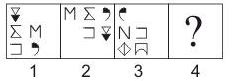RRB Group D Mock Test - 12 - Question 94

In the following problems, out of the five figures marked 1, 2, 3, 4, and 5, four are similar in a certain manner. However one figure is not like the other four. Choose the figure which is different from the rest.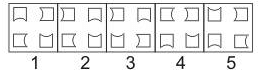Detailed Solution for RRB Group D Mock Test - 12 - Question 94

Only in fig. (3), two of the four elements are oriented in the same direction.
Hence, fig. (3) is the answer.

RRB Group D Mock Test - 12 - Question 95

In the following questions, select a figure from amongst the four alternatives, which when placed in the blank space of fig. (X) would complete the pattern.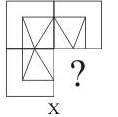RRB Group D Mock Test - 12 - Question 96

Eight friends A, B, C, D, E, F, G and H are sitting around a circle facing the centre. H is to the immediate left of E who is third to the right of B. C is second to the right of D and is not a neighbour of B. F is second to the right of G and is not a neighbour of C.

Q. Who is second to the right of E?

Detailed Solution for RRB Group D Mock Test - 12 - Question 96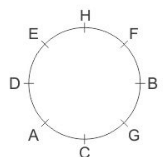RRB Group D Mock Test - 12 - Question 97

Find out from amongst the four alternatives as to how the pattern would appear when the transparent sheet is folded at the dotted line.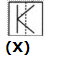RRB Group D Mock Test - 12 - Question 98

Answer these question based on the table given below .
The Hotel Company of India (HCI) owns seven Hotels with the same capacity. The occupancy rates across the seven hotels are given in the following table.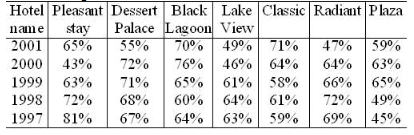Q. In which year did HCI witness the highest occupancy rate?

Detailed Solution for RRB Group D Mock Test - 12 - Question 98

Total occupancy for the year 1999 is the highest
2001 = 416, 2000 = 428,
1999 = 449, 1998 = 446, 1997 = 448

RRB Group D Mock Test - 12 - Question 99

Answer these question based on the table given below .
The Hotel Company of India (HCI) owns seven Hotels with the same capacity. The occupancy rates across the seven hotels are given in the following table.Q. Which one of the following statements is true?

RRB Group D Mock Test - 12 - Question 100

Answer these question based on the table given below .
The Hotel Company of India (HCI) owns seven Hotels with the same capacity. The occupancy rates across the seven hotels are given in the following table.Q. Which of the following statement (s) is/are false?

Detailed Solution for RRB Group D Mock Test - 12 - Question 100

Average occupancy rate hotel wise is given below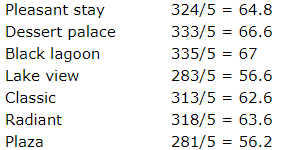From the above table it is given that all the statements given are false

## RRB (Group D) - Mock Tests & Previous Year Papers

1 docs|48 tests
 Use Code STAYHOME200 and get INR 200 additional OFF Use Coupon Code
Information about RRB Group D Mock Test - 12 Page
In this test you can find the Exam questions for RRB Group D Mock Test - 12 solved & explained in the simplest way possible. Besides giving Questions and answers for RRB Group D Mock Test - 12, EduRev gives you an ample number of Online tests for practice

1 docs|48 tests